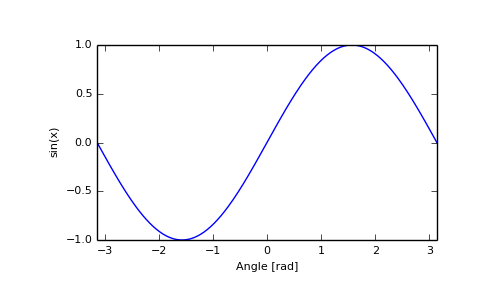# numpy.sin¶

numpy.sin(x[, out]) = <ufunc 'sin'>

Trigonometric sine, element-wise.

Parameters: x : array_like Angle, in radians (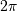rad equals 360 degrees). y : array_like The sine of each element of x.

Notes

The sine is one of the fundamental functions of trigonometry (the mathematical study of triangles). Consider a circle of radius 1 centered on the origin. A ray comes in from the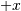axis, makes an angle at the origin (measured counter-clockwise from that axis), and departs from the origin. The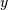coordinate of the outgoing ray’s intersection with the unit circle is the sine of that angle. It ranges from -1 for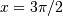to +1 for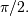The function has zeroes where the angle is a multiple of. Sines of angles betweenandare negative. The numerous properties of the sine and related functions are included in any standard trigonometry text.

Examples

Print sine of one angle:

>>> np.sin(np.pi/2.)
1.0


Print sines of an array of angles given in degrees:

>>> np.sin(np.array((0., 30., 45., 60., 90.)) * np.pi / 180. )
array([ 0.        ,  0.5       ,  0.70710678,  0.8660254 ,  1.        ])


Plot the sine function:

>>> import matplotlib.pylab as plt
>>> x = np.linspace(-np.pi, np.pi, 201)
>>> plt.plot(x, np.sin(x))